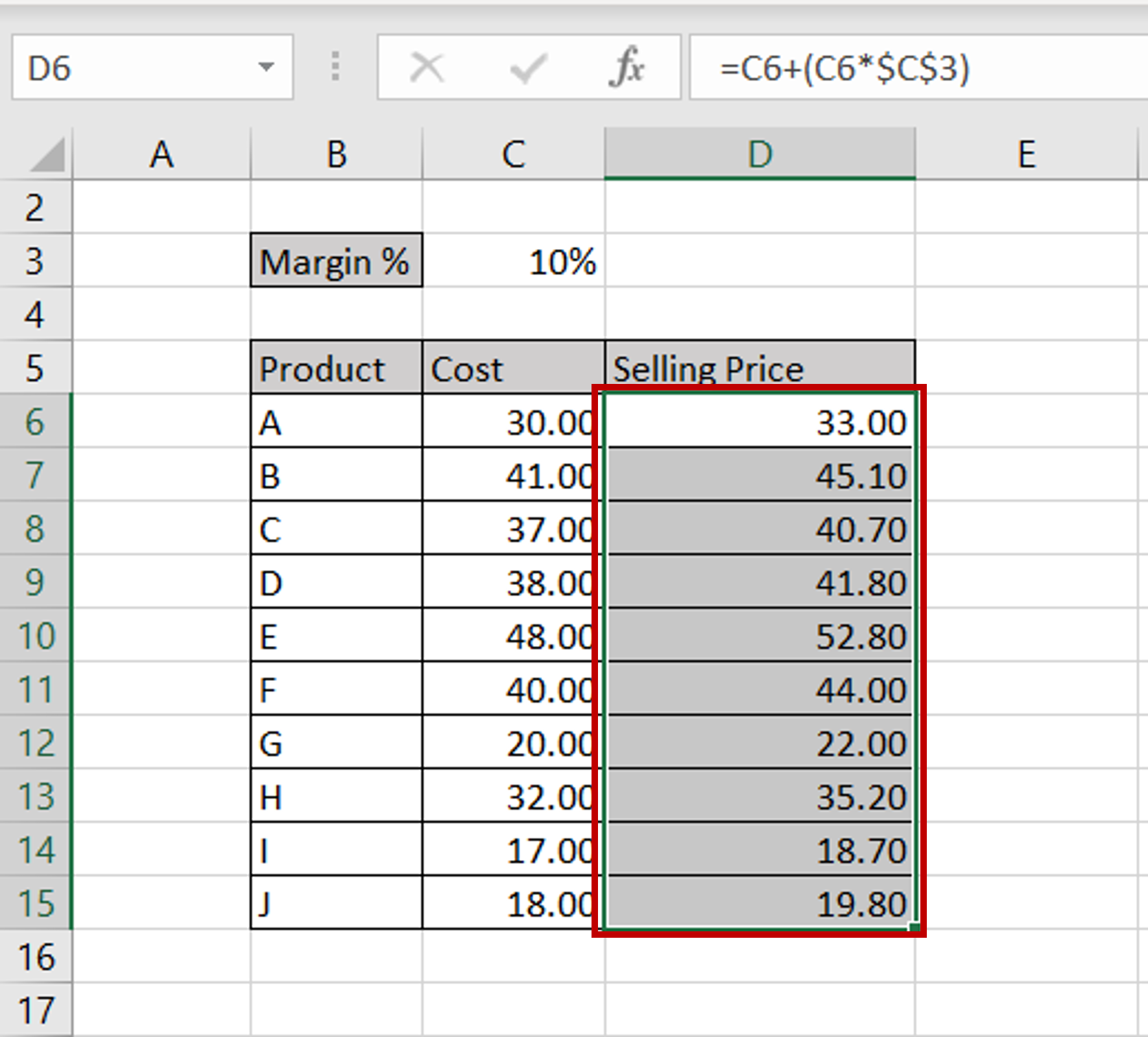# How to calculate selling price from cost and margin

You can watch a video tutorial here.Excel is frequently used for calculations and has many functions to help with basic mathematical operations.  When we have the cost and margin of a product, it is easy to compute the selling price using a simple formula in Excel. The formula is as follows:

Selling price = Cost + Margin %

The margin is a percentage of the cost so it changes according to the cost.

### Step 1 – Enter the formula– Select the destination cell
– Type the formula using cell references:
=Cost + (Margin% * Cost)
– Make the cell reference of the Margin % constant by selecting it and pressing F4 or by typing dollar signs in front of the row number and column header

### Step 2 – Copy the formula– Using the fill handle from the first cell, drag the formula to the remaining cells
OR
a) Select the cell with the formula and press Ctrl+C or choose Copy from the context menu (right-click)
b) Select the rest of the cells in the column and press Ctrl+V or choose Paste from the context menu (right-click)